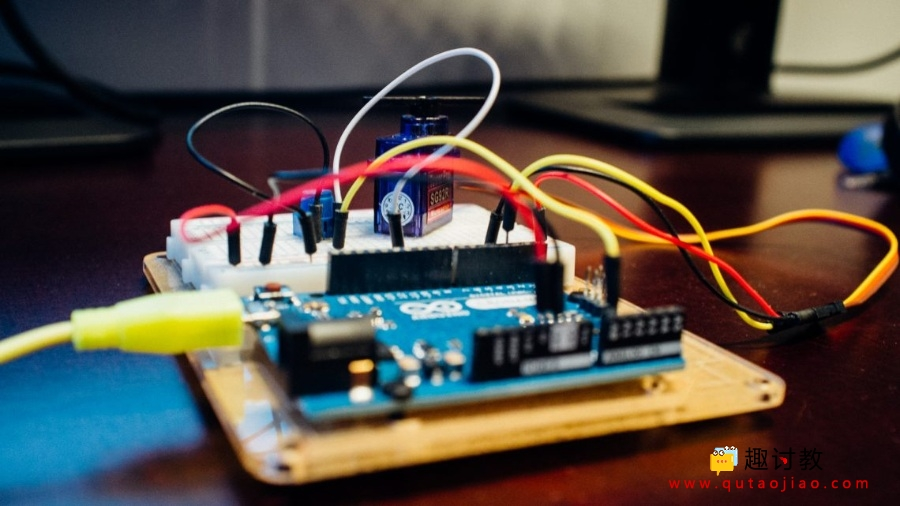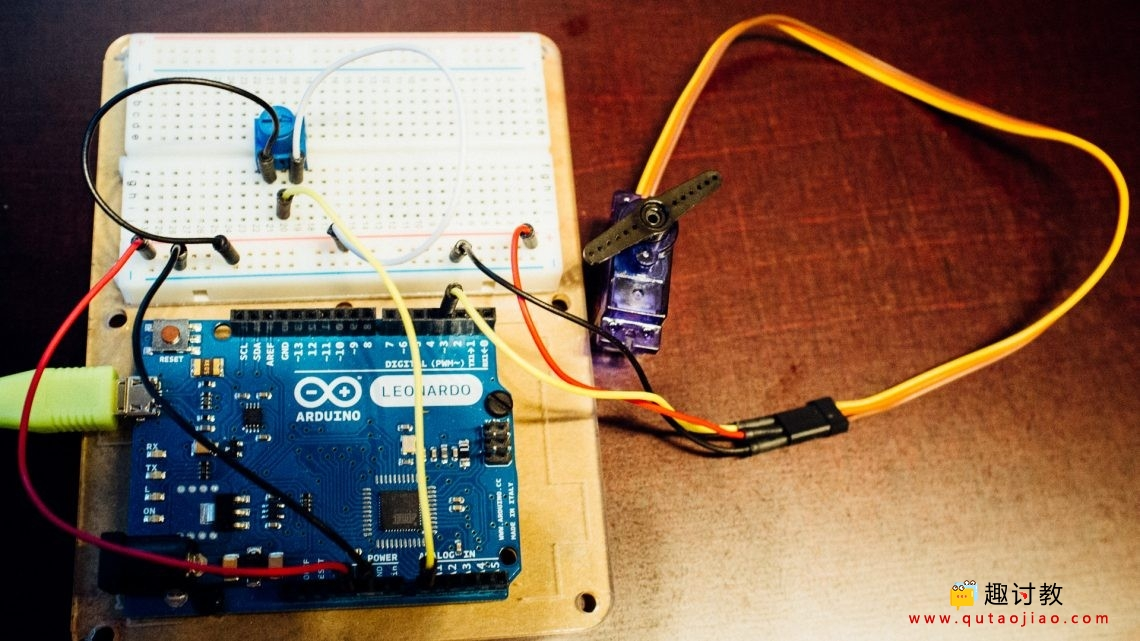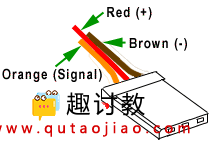# Arduino IDE串行绘图仪中的多个值

Arduino IDE1.6.7以上的版本，允许在串行绘图仪中绘制多个值。我们已经建立了一个示例，向您展示如何使用此功能。## 串行绘图器

Arduino的串行绘图器非常易于使用。串行绘图实际上不像串行监视器那样显示一堆数字和/或字符，而是随着时间推移绘制数字，其中y轴是值，x轴是时间。这将生成一个实时绘制的任何数字变量的图形。

y轴具有简单的自动缩放功能，可适应这些值，而x轴则显示最后x条线（即样本）。主循环中的20ms延迟使串行绘图仪显示约。数据的最后15秒（循环本身没有太多处理时间）。增加此延迟（即减少打印频率）以减慢滚动x轴的速度。

## 我们的例子```#include

Servo myservo;          //create servo object

//Global Variables
int sensorPin = 0;      //pin number to use the ADC
int sensorValue = 0;    //initialization of sensor variable, equivalent to EMA Y
float EMA_a = 0.06;     //initialization of EMA alpha
int EMA_S = 0;          //initialization of EMA S
int EMA_S_map = 0;      //initialization of variable for servo control

void setup(){
Serial.begin(115200);               //setup of Serial module, 115200 bits/second
EMA_S = analogRead(sensorPin);      //set EMA S for t=1
myservo.attach(3);                  //servo initialization
}

void loop(){
EMA_S = (EMA_a*sensorValue) + ((1-EMA_a)*EMA_S);    //run the EMA

Serial.print(sensorValue);      //the first variable for plotting
Serial.print(",");              //seperator
Serial.println(EMA_S);          //the second variable for plotting including line break

EMA_S_map = map(EMA_S, 0, 1023, 0, 180);    //map ADC values to servo values (0-180)
myservo.write(EMA_S_map);                   //send the latest value to the servo

delay(20);          //20ms delay
}
```EMA_a = 0.06。蓝色：原始值（电位计），橙色：滤波值（伺服）EMA_a = 0.3。与上图相同的时间跨度，但响应速度更快。

## Basic Techniques

2019-11-1 18:50:21

## STC8低功耗总结

2019-11-15 20:05:16

0 条回复 A文章作者 M管理员
暂无讨论，说说你的看法吧